# FREQUENCY SAMPLING FILTERS: THE LOST ART

### FREQUENCY SAMPLING FILTERS THE LOST ART

This section describes a class of digital filters, called frequency sampling filters, used to implement linear-phase FIR filter designs. Although frequency sampling filters were developed over 35 years ago, the advent of the powerful Parks-McClellan nonrecursive FIR filter design method has driven them to near obscurity. In the 1970s, frequency sampling filter implementations lost favor to the point where their coverage in today's DSP classrooms and textbooks ranges from very brief to nonexistent. However, we'll show how frequency sampling filters remain more computationally efficient than Parks-McClellan–designed filters for certain applications where the desired passband width is less than roughly one fifth the sample rate. The purpose of this material is to introduce the DSP practitioner to the structure, performance, and design of frequency sampling filters; and to present a detailed comparison between a proposed high-performance frequency sampling filter implementation and its nonrecursive FIR filter equivalent. In addition, we'll clarify and expand the literature of frequency sampling filters concerning the practical issues of phase linearity, filter stability, gain normalization, and computational workload using design examples.

Frequency sampling filters were founded upon the fact that the traditional N-tap nonrecursive (direct convolution) FIR filter in Figure 7-1(a) can be implemented as a comb filter in cascade with a bank of N complex resonators as shown in Figure 7-1(b). We call the filter in Figure 7-1(b) a general frequency sampling filter (FSF), and its equivalence to the nonrecursive FIR filter has been verified[1–3]. While the h(k) coefficients, where 0 < k < N–1, of N-tap nonrecursive FIR filters are typically real-valued, in general they can be complex. That's the initial assumption made in equating the two filters in Figure 7-1. The H(k) gain factors, the discrete Fourier transform of the h(k) time-domain coefficients, are in the general case complex values represented by |H(k)|ejø(k).

Figure 7-1. FIR filters: (a) N-tap nonrecursive; (b) equivalent N-section frequency sampling filter.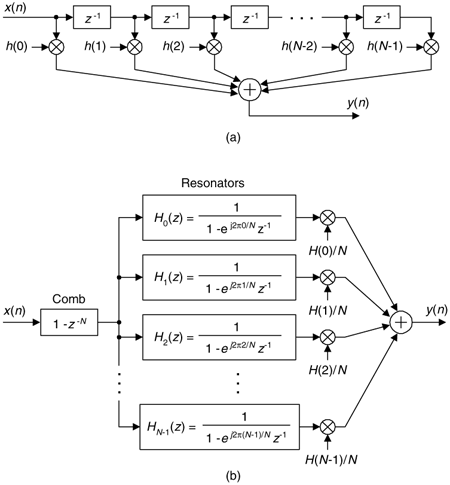The basis of FSF design is the definition of a desired FIR filter frequency response in the form of H(k) frequency-domain samples, whose magnitudes are depicted as dots in Figure 7-2. Next, those complex H(k) sample values as used as gain factors following the resonators in the FSF structure (block diagram). If you haven't seen it before, please don't be intimidated by this apparently complicated FSF structure. We'll soon understand every part of it, and how those parts work together.

Figure 7-2. Defining a desired filter response by frequency sampling.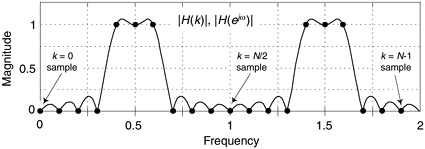Later we'll develop the math to determine the interpolated (actual) frequency magnitude response |H(ejw)| of an FSF shown by the continuous curve in Figure 7-2. In this figure, the frequency axis labeling convention is a normalized angle measured in p radians with the depicted w frequency range covering 0 to 2p radians, corresponding to a cyclic frequency range of 0 to fs, where fs is the sample rate in Hz.

To avoid confusion, we remind the reader there is a popular nonrecursive FIR filter design technique known as the frequency sampling design method described in the DSP literature. That design scheme begins (in a manner similar to an FSF design) with the definition of desired H(k) frequency response samples, then an inverse discrete Fourier transform is performed on those samples to obtain a time-domain impulse response sequence that's used as the h(k) coefficients in the nonrecursive N-tap FIR structure of Figure 7-1(a). In the FSF design method described here, the desired frequency-domain H(k) sample values are the coefficients used in the FSF structure of Figure 7-1(b), which is typically called the frequency sampling implementation of an FIR filter.

Although more complicated than nonrecursive FIR filters, FSFs deserve study because in many narrowband filtering situations they can implement a linear-phase FIR filter at a reduced computational workload relative to an N-tap nonrecursive FIR filter. The computation reduction occurs because, while all of the h(k) coefficients are used in the nonrecursive FIR filter implementation, most of the H(k) values will be zero-valued, corresponding to the stopband, and need not be implemented. To understand the function and benefits of FSFs, we start by considering the behavior of the comb filter and then review the performance of a single digital resonator.

7.1.1 A Comb Filter and Complex Digital Resonator in Cascade

A single section of a complex FSF is a comb filter followed by a single complex digital resonator as shown in Figure 7-3.

Figure 7-3. A single section of a complex FSF.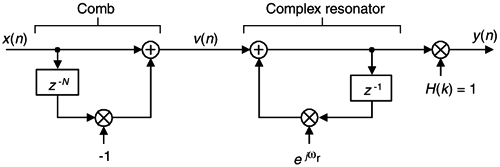The 1/N gain factor following a resonator in Figure 7-1(b) is omitted, for simplicity, from the single-section complex FSF. (The effect of including the 1/N factor will be discussed later.) To understand the single-section FSF's operation, we first review the characteristics of the nonrecursive comb filter whose time-domain difference equation is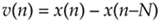with its output equal to the input sequence minus the input delayed by N samples. A single-section FSF z-domain transfer function is

Equation 7-2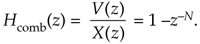The frequency response of a comb filter, derived in Appendix G, Section 1, is

Equation 7-3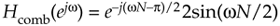with a magnitude response of |Hcomb(ejw)| = 2|sin(wN/2)| whose maximum value is 2. It's meaningful to view the comb filter's time-domain impulse response and frequency-domain magnitude response shown in Figure 7-4 for N = 8. The magnitude response makes it clear why the term "comb" is used.

Figure 7-4. Time and frequency-domain characteristics of an N = 8 comb filter.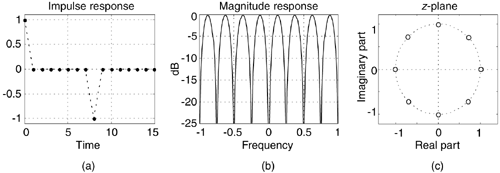Equation (7-2) leads to a key feature of this comb filter: its transfer function has N periodically spaced zeros around the z-plane's unit circle shown in Figure 7-4(c). Each of those zeros, located at z(k) = ej2pk/N, where k = 0, 1, 2, . . ., N–1, corresponds to a magnitude null in Figure 7-4(b), where the normalized frequency axis is labeled from –p to +p radians. Those z(k) values are the N roots of unity when we set Eq. (7-2) equal to zero yielding z(k)N = (ej2pk/N)N = 1. We can combine the magnitude response (on a linear scale) and z-plane information in the three-dimensional z-plane depiction shown in Figure 7-5, where we see the intersection of the |Hcomb(z)| surface and the unit circle. Breaking the curve at the z = –1 point, and laying it flat, corresponds to the magnitude curve in Figure 7-4(b).

Figure 7-5. The z-plane frequency magnitude response of the N = 8 comb filter.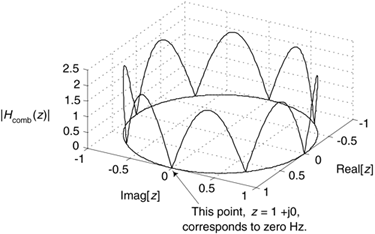To preview where we're going, soon we'll build an FSF by cascading the comb filter with a digital resonator having a transfer function pole lying on top of one of the comb's z-plane zeros, resulting in a linear-phase bandpass filter. With this thought in mind, let's characterize the digital resonator in Figure 7-3.

The complex resonator's time-domain difference equation is

Equation 7-4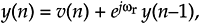where the angle wr, -p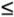wrp determines the resonant frequency of our resonator. We show this by considering the resonator's z-domain transfer function

Equation 7-5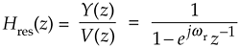and the resonator's complex time-domain impulse response, for wr = p/4, in Figure 7-6.

Figure 7-6. Single complex digital resonator impulse response with wr = p/4.The wr = p/4 resonator's impulse response is a complex sinusoid, the real part (a cosine sequence) of which is plotted in Figure 7-7(a), and can be considered infinite in duration. (The imaginary part of the impulse response is, as we would expect, a sinewave sequence.) The frequency magnitude response is very narrow and centered at wr. The resonator's Hres(z) has a single zero at z = 0, but what concerns us most is its pole, at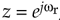, on the unit circle at an angle of wr as shown in Figure 7-7(c). We can think of the resonator as an infinite impulse response (IIR) filter that's conditionally stable because its pole is neither inside nor outside the unit circle.

Figure 7-7. Time and frequency-domain characteristics of a single complex digital resonator with wr = p/4.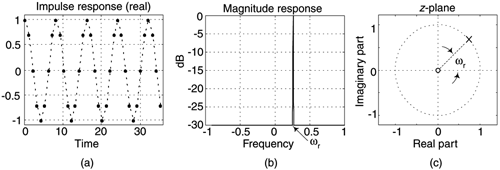We now analyze the single-section complex FSF in Figure 7-3. The z-domain transfer function of this FSF is the product of the individual transfer functions and H(k), or

Equation 7-6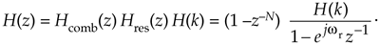If we restrict the resonator's resonant frequency wr to be 2pk/N, where k = 0, 1, 2, . . ., N–1, then the resonator's z-domain pole will be located atop one of the comb's zeros and we'll have an FSF transfer function of

Equation 7-7where the subscript "ss" in Eq. (7-7) means a single-section complex FSF. We can understand a single-section FSF by reviewing its time and frequency-domain behavior for N = 32, k = 2, and H(2) = 1 shown in Figure 7-8.

Figure 7-8. Time and frequency-domain characteristics of a single-section complex FSF where N = 32, k = 2, and H(2) = 1.Figure 7-8 is rich in information. We see that the complex FSF's impulse response is a truncated complex sinusoid whose real part is shown in Figure 7-8(a). The positive impulse from the comb filter started the resonator oscillation at zero time. Then at just the right sample, N = 32 samples later—which is k = 2 cycles of the sinusoid—the negative impulse from the comb arrives at the resonator to cancel all further oscillation. The frequency magnitude response, being the Fourier transform of the truncated sinusoidal impulse response, takes the form of a sin(x)/x-like function. In the z-plane plot of Figure 7-8, the resonator's pole is indeed located atop the comb filter's k = 2 zero on the unit circle canceling the frequency magnitude response null at 2pk/N = p/8 radians. (Let's remind ourselves that a normalized angular frequency of 2pk/N radians corresponds to a cyclic frequency of kfs/N where fs is the sample rate in Hz. Thus the filter in Figure 7-8 resonates at fs/16 Hz.)

We can determine the FSF's interpolated frequency response by evaluating the Hss(z) transfer function on the unit circle. Substituting ejw for z in Hss(z) in Eq. (7-7), as detailed Appendix G, Section 2, we obtain an Hss(ejw) frequency response of

Equation 7-8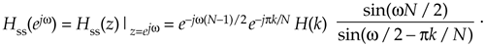Evaluating |Hss(ejw)| over the frequency range of –p < w < p yields the curve in Figure 7-8(b). Our single-section FSF has linear phase because the e–jpk/N term in Eq. (7-8) is a fixed phase angle based on constants N and k, the angle of H(k) is fixed, and the e–jw(N–1)/2 phase term is a linear function of frequency (w). As derived in Appendix G, Section 2, the maximum magnitude response of a single-section complex FSF is N when |H(k)| = 1. We illustrate this fact in Figure 7-9.

Figure 7-9. The z-plane frequency magnitude response of a single-section complex FSF with N = 32 and k = 2.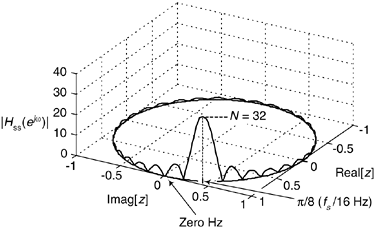7.1.2 Multisection Complex FSFs

In order to build useful FSFs we use multiple resonator sections, as indicated in Figure 7-1(b), to provide bandpass FIR filtering. For example, let's build a three-section complex bandpass FSF by establishing the parameters of N = 32 with the non-zero frequency samples H(2), H(3), and H(4). The desired frequency magnitude response is shown in Figure 7-10(a) with the bandpass FSF structure provided in Figure 7-10(b).

Figure 7-10. Three-section N = 32 complex FSF: (a) desired frequency magnitude response; (b) implementation.Exploring this scenario, recall that the z-domain transfer function of parallel filters is the sum of the individual transfer functions. So, the transfer function of an N-section complex FSF from Eq. (7-7) is

Equation 7-9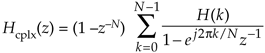where the subscript "cplx" means a complex multisection FSF.

Let's pause for a moment to understand Eq. (7-9). The first factor on the right side represents a comb filter, and the comb is in cascade (multiplication) with the sum of ratio terms. The summation of the ratios (each ratio is a resonator) means those resonators are connected in parallel. Recall from Section 6.8.1 that the combined transfer function of filters connected in parallel is the sum of the individual transfer functions. It's important to be comfortable with the form of Eq. (7-9) because we'll be seeing many similar expressions in the material to come.

So a comb filter is driving a bank of resonators. For an N = 32 complex FSF we could have up to 32 resonators, but in practice only a few resonators are needed for narrowband filters. In Figure 7-10, we used only three resonators. That's the beauty of FSFs: most of the H(k) gain values in Eq. (7-9) are zero-valued and those resonators are not implemented, keeping the FSF computationally efficient.

Using the same steps as in Appendix G, Section 2, we can write the frequency response of a multisection complex FSF, such as in Figure 7-10, as

Equation 7-10

[Image_Link]/"bookImages/">Amazon